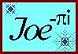SLOPED RIDGE DORMER CALCULATIONS

 Animated Graphics Revolution of Deck or Reference Plane Changing the Slope of the Dormer Ridge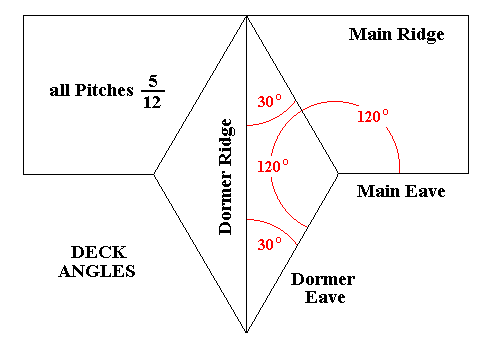Deck Angles or Plan Angles

Solving the Deck Angles or Plan Angles :
 The Dormer Ridge is equivalent to a Hip Rafter Ridge or Valley Rafter Trough Hip-Valley Pitch = Common Pitch sin Deck Angle Therefore sin Deck Angle = Hip-Valley Pitch ÷ Common Pitch and Deck Angle = arcsin (Hip-Valley Pitch ÷ Common Pitch) = arcsin (2.5 ÷ 5) = arcsin (.5) = 30° The Total Deck Angle between the Dormer Eaves = 2 × 30° = 60° The Total Deck Angle between the Dormer Eave and the Main Eave = 240°

Lag Calculator Entries :
5 ÷ 12 , 5 ÷ 12 , 60°

 Click on or button (equal Common Pitches; Main Side = Adjacent Side) SS = Common Pitch Angle = 22.619865° DD = Deck Angle or Plan Angle = 30.000000° R1 = Hip-Valley Pitch Angle = 11.768289° (= 2 1/2 ÷ 12 , as required) 90° - P2 = Angle on Roof Plane between Dormer Eave and Dormer Ridge = 32.024447° C5 = Backing Angle = 19.456233°

Lag Calculator Entries :
5 ÷ 12 , 5 ÷ 12 , 240°

 Click on or button (equal Common Pitches; Main Side = Adjacent Side) SS = Common Pitch Angle = 22.619865° DD = Deck Angle or Plan Angle = 120.000000° R1 = Hip-Valley Pitch Angle = 19.841670° (= 4 11/32 ÷ 12) 90° - P2 = Angle on Roof Plane between Valley Trough and Main Ridge = 61.945119° C5 = Backing Angle = 11.087489°

Angles on the Triangle of the Dormer Roof Plane :
 Angle on Roof Plane between Valley Trough and Main Eave = 180° - 61.945119° = 118.054881° Invoking symmetry: Angle on Roof Plane between Valley Trough and Dormer Ridge = 180° - (118.054881° + 32.024447°) = 29.920672°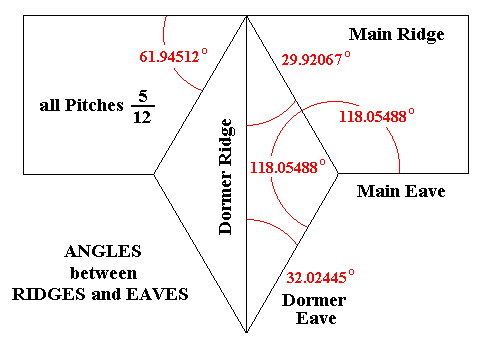Angles between Ridges and Eaves

Checking the Angles on the Roof Planes :
 For the following calculations the plane of the Total Deck Angle or Plan Angle, the level plane through the Main Eave and Dormer Eave, is rotated until it contacts the Main Ridge and Dormer Ridge creating a REFERENCE PLANE for subsequent calculations. The Total Deck Angle remains 90°, bounded by the line of the Main Ridge and the line of the Dormer Ridge. The Main "Pitch Angle" with respect to the rotated "Deck" = 22.619865° - 11.768292° = 10.851576° (refer to the Section View and 3D Sketch below) The Adjacent "Pitch Angle" with respect to the rotated "Deck" is NOT 22.619865° as it might seem at first glance. Rather, the dihedral angle required here is the Backing Angle 19.456233° returned by the initial Lag Program calculation. In other words, the "Pitch Angle" is the angle required to bevel the Dormer Ridge which is in essence a Hip Rafter, with the REFERENCE PLANE following the upper shoulder. (refer to the 3D Sketch detailing boundaries of REFERENCE PLANE)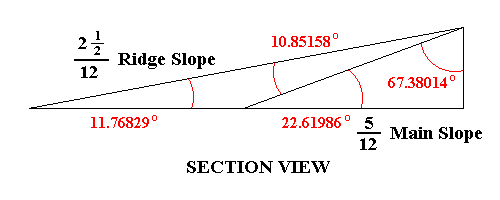Section through 5 ÷ 12 Main Roof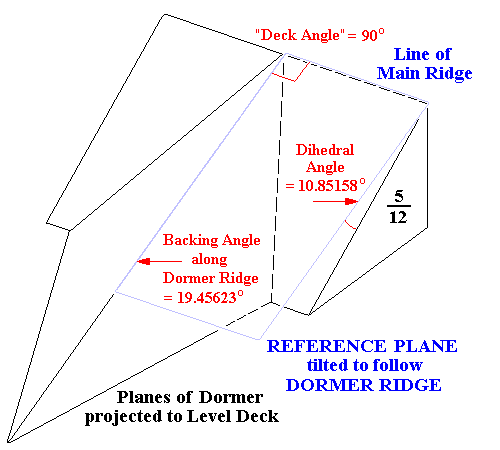3D Sketch detailing boundaries of REFERENCE PLANE

LAG Calculator Entries (select Angle Mode) :
 MAIN PITCH Rise : 10.851576° ADJACENT PITCH Rise : 19.456233° Total Deck Angle : 90° (Disregard the Run fields in Angle Mode)
 Select 90 -P2 : Roof plane from drop-down menu, click on button 90° - P2m = Angle on Roof Plane between Valley Trough and Main Ridge = 61.94512° (Compare this result to the return from the second program calculation) Select 90 -P2 : Roof plane from drop-down menu, click on button 90° - P2a = Angle on Roof Plane between Valley Trough and Dormer Ridge = 29.92067° (Compare this result to the calculation for Angles on the Triangle of the Dormer Roof Plane)

Rotating the REFERENCE PLANE to the 5 ÷ 12 Roof Plane :
 From the second Lag Program calculation: C5 = Backing Angle = 11.087489° "Pitch Angle" of the plane of the dormer with respect to the plane of the 5 ÷ 12 Main Roof = 2 × 11.087489° = 22.174978° 90° - P2 = Angle on Roof Plane between Valley Trough and Main Ridge = 61.945119° "Deck Angle" between the Valley Trough lines on the 5 ÷ 12 Main Roof = 180° - (2 × 61.945119°) = 56.109762°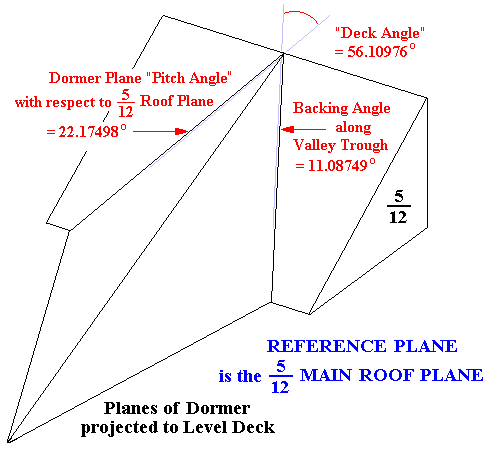3D Sketch : the 5 ÷ 12 Main Roof Plane is the REFERENCE PLANE

LAG Calculator Entries (select Angle Mode) :
 MAIN PITCH Rise : 22.174978° ADJACENT PITCH Rise : 22.174978° Total Deck Angle : 56.109762° (Disregard the Run fields in Angle Mode)
 Select 90 -P2 : Roof plane from drop-down menu Click on or button (equal Common Pitches; Main Side = Adjacent Side) 90° - P2 = Angle on Roof Plane between Valley Trough and Dormer Ridge = 29.92067° (Compare this result to the previous calculations for this angle) Angle of the Dormer Ridge with respect to the 5 ÷ 12 Roof Plane : Select R1 : Hip / Valley rafter from drop-down menu Click on or button R1 = Hip-Valley Pitch Angle = 10.85158° , as per the Section View above Different program entry values, different reference planes and manual calculations were employed throughout this process, and all the results still agree to five decimal places. The image below details the Angles between Ridges and Eaves and dimensions based on a Main Run = 10.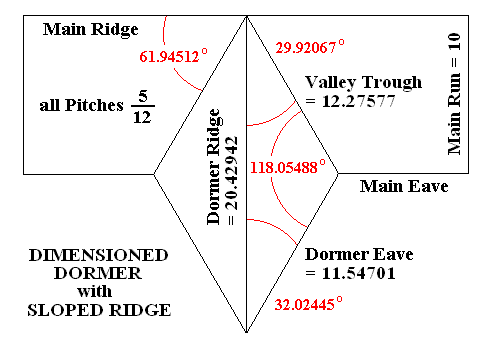Eave and Ridge dimensions based on Main Run = 10

 If the actual dimensions are not known the scale can be set with respect a Unit Length as per the sketch below. The ratios may be re-scaled later to produce dimensions.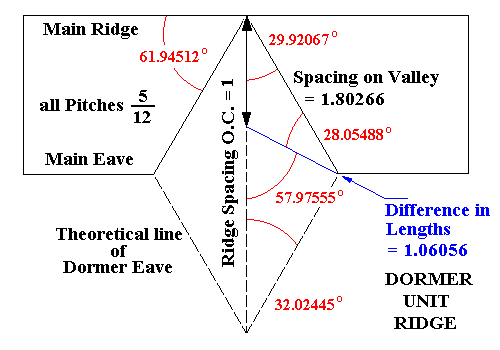Spacing and Difference in Length Unit Factors
Angles depicted are on the planes of the Hip-Valley Roof

Applying the Unit Difference in Length Ratios :
 All of the ratios may be multiplied by the same factor to yield dimensions in terms of the actual roof. For example, if the Spacing O.C. along the Dormer Ridge = 16" : Jack Rafter Difference in Length = 16" × 1.06056 = 16.96896" = 16 31/32" Spacing along Valley Rafter = 16" × 1.80266 = 28.84256" = 28 27/32" Another example: If the distance along the Dormer Ridge to a Jack Rafter = 48" : Jack Rafter Length = 48" × 1.06056 = 50.90688" = 50 29/32" Dimension along Valley Rafter = 48" × 1.80266 = 86.52768" = 86 17/32"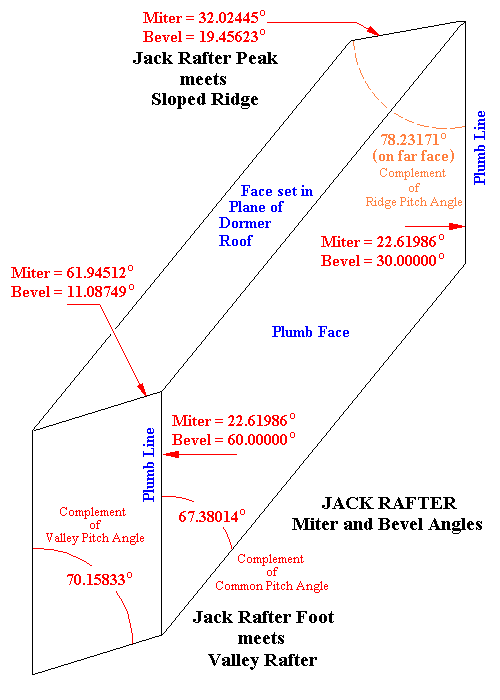Jack Rafter Miter and Bevel Angles
The Compound Angle is the same at the 5 ÷ 12 Main Roof Rafter Feet

Checking the Compound Angles :

Dropping the Ridge :
 The Dormer Ridge is a Hip Rafter and must be dropped if the Jack Rafters are to plane correctly. Ridge Drop = Half of Ridge Width × tan Plumb Backing Angle Ridge Drop = .750000" × tan 19.841670° = .270633" (approx. = 1/4")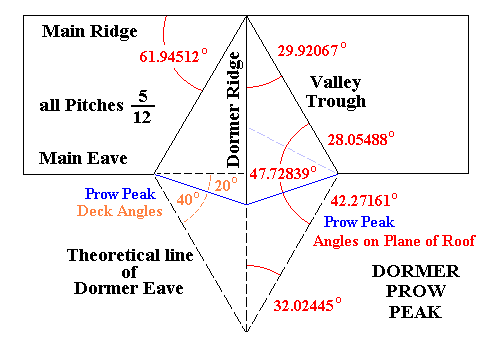Prow Peak detailing Deck Angles (left)
and Angles on the Plane of the Roof (right)

Example Prow Peak Calculations :
 The Prow Peak is treated as a Valley Rafter. The givens may be a dimension, the Prow Peak Pitch, or the Deck Angle; for the purpose of the following example calculation a value of 20° will be assigned at the Prow Peak creating a 40° Deck Angle. Prow Peak Pitch Angle = arctan (tan Common Pitch Angle sin Deck Angle) Prow Peak Pitch Angle = arctan (tan 22.619865° sin 40.000000°) = 14.993530° (= 3 7/32 ÷ 12) The Angle on the Plane of the Roof at the foot of the Prow Peak = arctan (tan Deck Angle ÷ cos Common Pitch Angle) = = arctan (tan 40.000000° ÷ cos 22.619865°) = 42.271609° The Jack Rafters originate at right angles to the Main Ridge and the theoretical line of the Dormer Eave projected to the deck or level (refer to the diagram below). Therefore they intercept the Prow Peak at 90° - 42.271609° = 47.728391° Sum of the Angles between the Valley Trough and the Dormer Eave = 42.271609° + 47.728391° + 28.054881° = 118.054881° (as per previous calculations) Compound Angle at Jack Rafter meets Prow Peak : Angle on the Plumb Face meets Side Face of Prow Peak = 90° - 14.993530° = 75.006470° Jack Rafter Plumb Cut = arcsin (cos 75.006470° ÷ cos 47.728391°) = 22.619865° (the correct Pitch Angle for 5 ÷ 12) Saw Blade Bevel = arctan (cos 22.619865° tan 42.271609°) = 40.000000° (the specified Deck Angle) Saw Blade Bevel along the 47.728391° line (Miter Angle = 42.271609°) on the Roof Plane = arctan (cos 42.271609° tan 22.619865°) = 17.135500° Treating the Prow Peak as a Valley Rafter, the Backing Angle may be solved by the formula: Backing Angle = arctan (sin Hip-Valley Pitch Angle ÷ tan Deck Angle) Backing Angle = arctan (sin 14.993530° ÷ tan 40.000000°) = 17.135500° (confirming the calculation for the Saw Blade Bevel along the line on the Roof Plane) A set of ratios with respect to any convenient Unit Length may be calculated for the 57.975553° : 47.728391° : 74.296065° triangle at the Prow Peak. Setting the Dormer Ridge = 1.00000 Jack Rafter Difference in Length = 1.30097 Spacing along Prow Peak = 1.14576 If the Spacing O.C. along the Dormer Ridge = 16" Jack Rafter Difference in Length = 16" × 1.30097 = 20.81552" = 20 13/16" Spacing along Prow Peak = 16" × 1.14576 = 18.33216" = 18 11/32"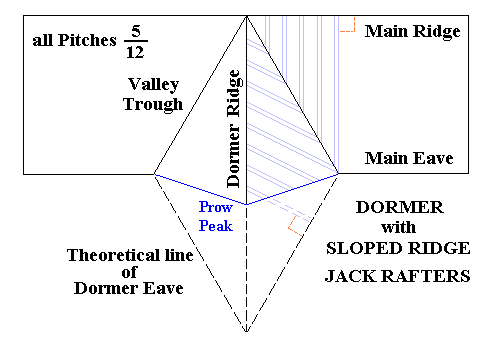Prow Peak showing orientation of Jack Rafters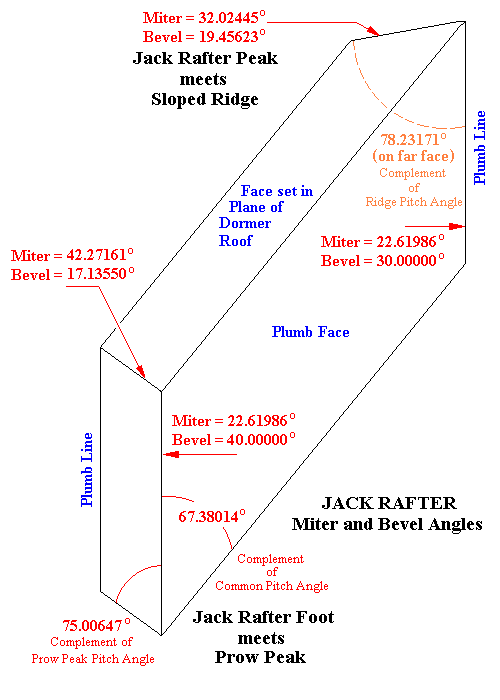Compound Angle at Jack Rafter Foot meets Prow Peak
Jack Rafter Peak meets Sloped Ridge remains the same